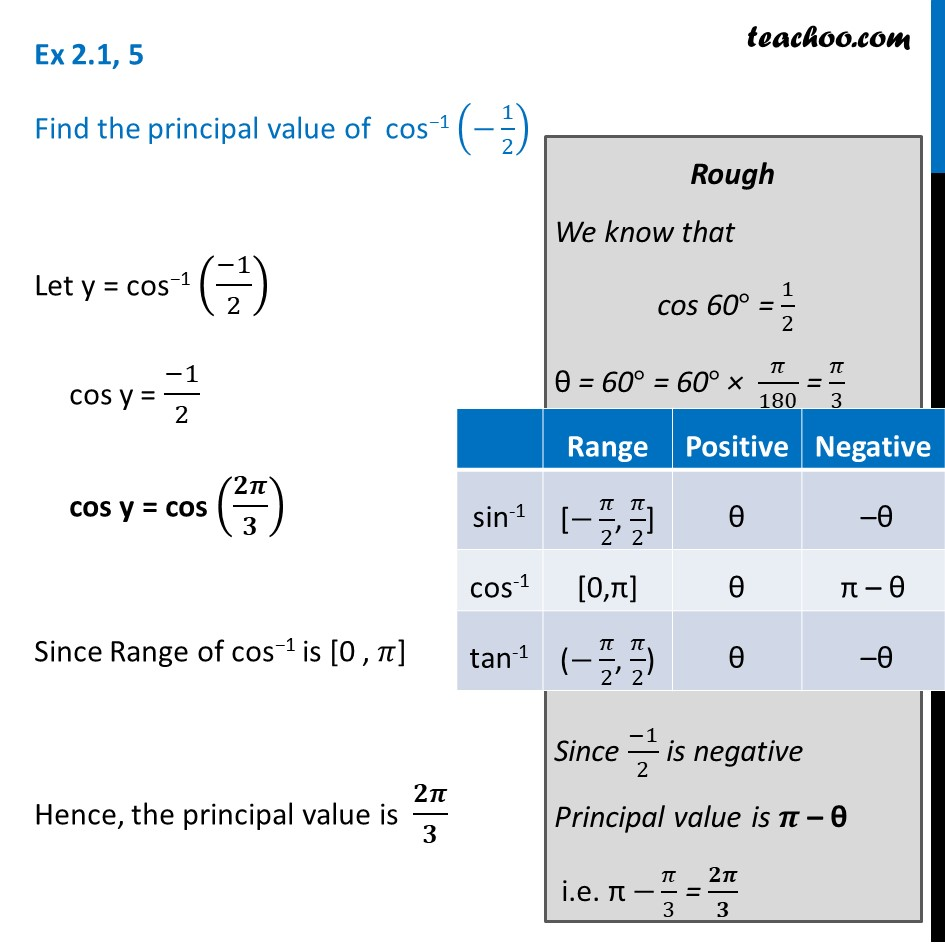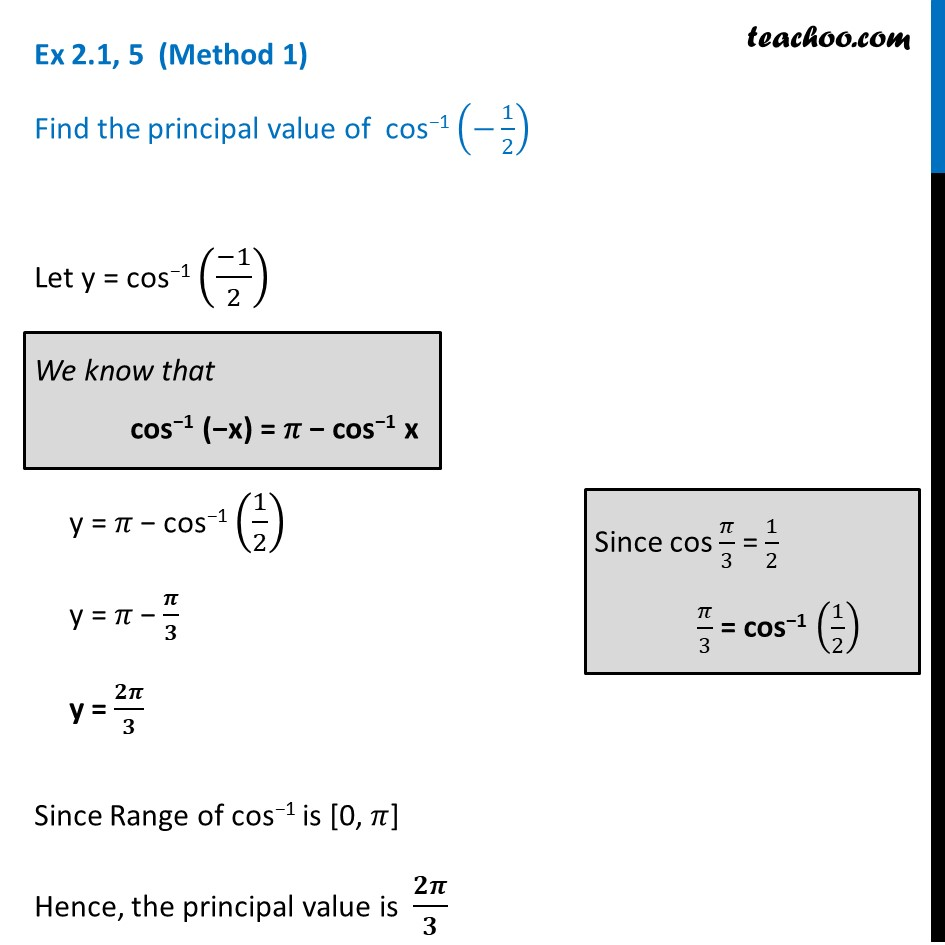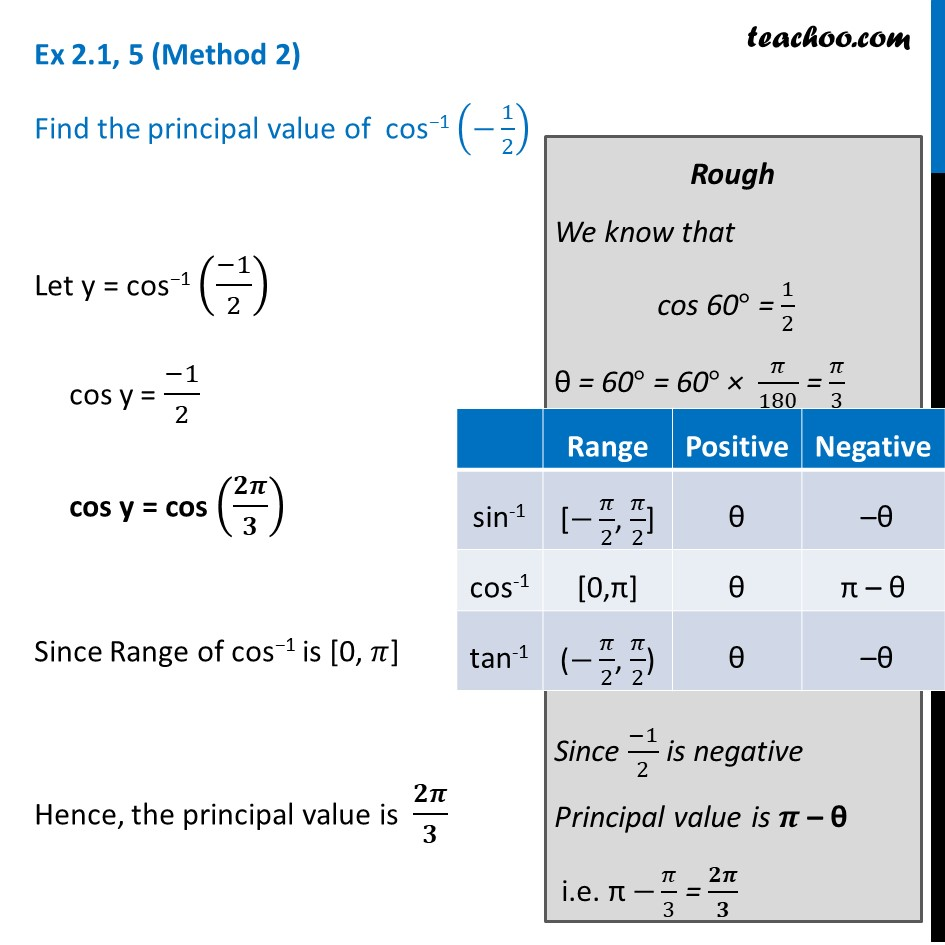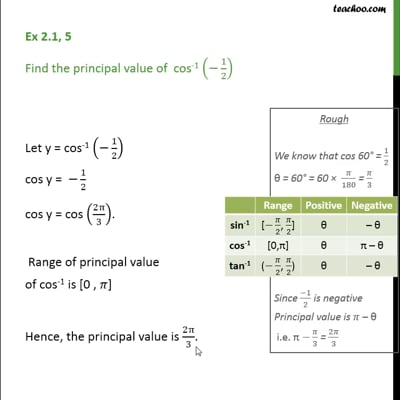Chapter 2 Class 12 Inverse Trigonometric Functions

Class 12
Important Questions for exams Class 12This video is only available for Teachoo black users

Solve all your doubts with Teachoo Black (new monthly pack available now!)

### Transcript

Ex 2.1, 5 Find the principal value of cos−1 (−1/2) Let y = cos−1 ((−1)/2) cos y = (−1)/2 cos y = cos (𝟐𝝅/𝟑) Since Range of cos−1 is [0 , 𝜋] Hence, the principal value is 𝟐𝝅/𝟑 Rough We know that cos 60° = 1/2 θ = 60° = 60° × 𝜋/180 = 𝜋/3 Since (−1)/2 is negative Principal value is 𝝅 – θ i.e. π −𝜋/3 = 𝟐𝝅/𝟑 Ex 2.1, 5 (Method 1) Find the principal value of cos−1 (−1/2) Let y = cos−1 ((−1)/2) y = 𝜋 − cos−1 (1/2) y = 𝜋 − 𝝅/𝟑 y = 𝟐𝝅/𝟑 Since Range of cos−1 is [0, 𝜋] Hence, the principal value is 𝟐𝝅/𝟑 We know that cos−1 (−x) = 𝜋 − cos−1 x Since cos 𝜋/3 = 1/2 𝜋/3 = cos−1 (1/2) Ex 2.1, 5 (Method 2) Find the principal value of cos−1 (−1/2) Let y = cos−1 ((−1)/2) cos y = (−1)/2 cos y = cos (𝟐𝝅/𝟑) Since Range of cos−1 is [0, 𝜋] Hence, the principal value is 𝟐𝝅/𝟑 Rough We know that cos 60° = 1/2 θ = 60° = 60° × 𝜋/180 = 𝜋/3 Since (−1)/2 is negative Principal value is 𝝅 – θ i.e. π −𝜋/3 = 𝟐𝝅/𝟑